This thread has been locked.

If you have a related question, please click the "Ask a related question" button in the top right corner. The newly created question will be automatically linked to this question.

# TINA/Spice/OPA375: Using TINA to simulate the influence of CMRR and PSRR of OPA735

Part Number: OPA375
Other Parts Discussed in Thread: TINA-TI, OPA735

Tool/software: TINA-TI or Spice Models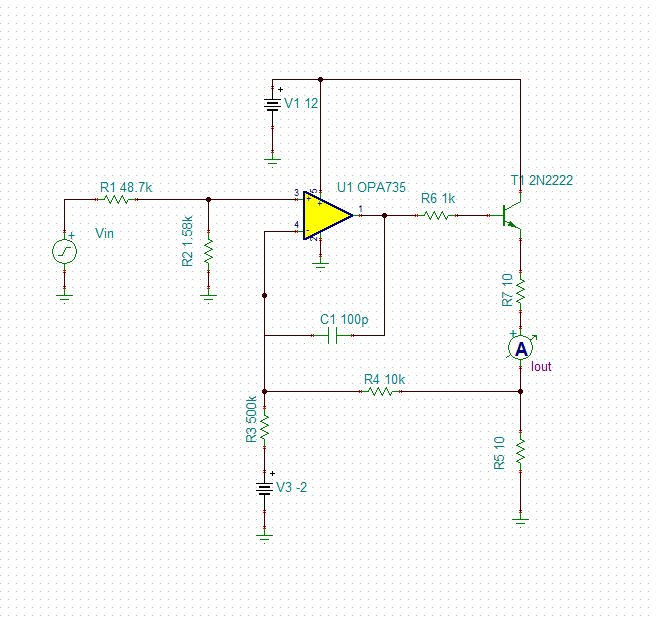The above circuit is a V-I circiut, the input voltage is 0-5V and the output current is 4-20mA. The amplifier is OPA735 and the NPN bipolar transistor is 2N2222.

When simulating the influence of CMRR on the above circuit, when adding a 100mV small sine wave in the input DC voltage source, the wave of output current trend to decrease with the increasing of the frequency of the small sine wave adding in the  voltage source.

For example, when the frequency of small sine wave is 50KHz, the wave of output current is 0.3mA and when the frequency is 500kHz,the wave of output current is under 0.2mA. The simulation results are shown as follow, why the output current wave decreas with the increasing of frequency.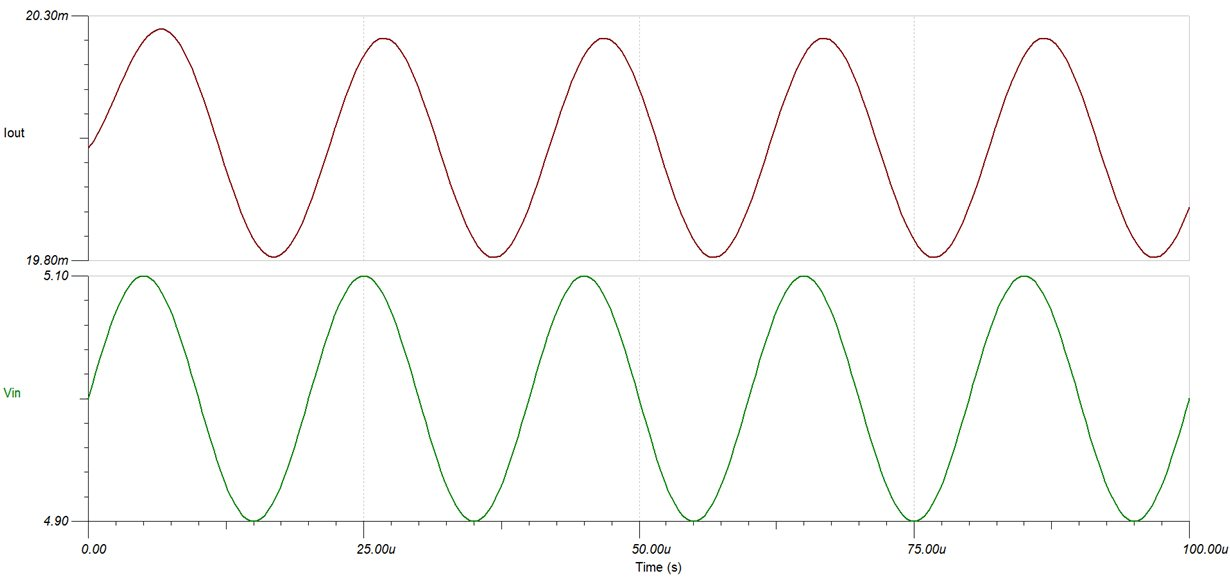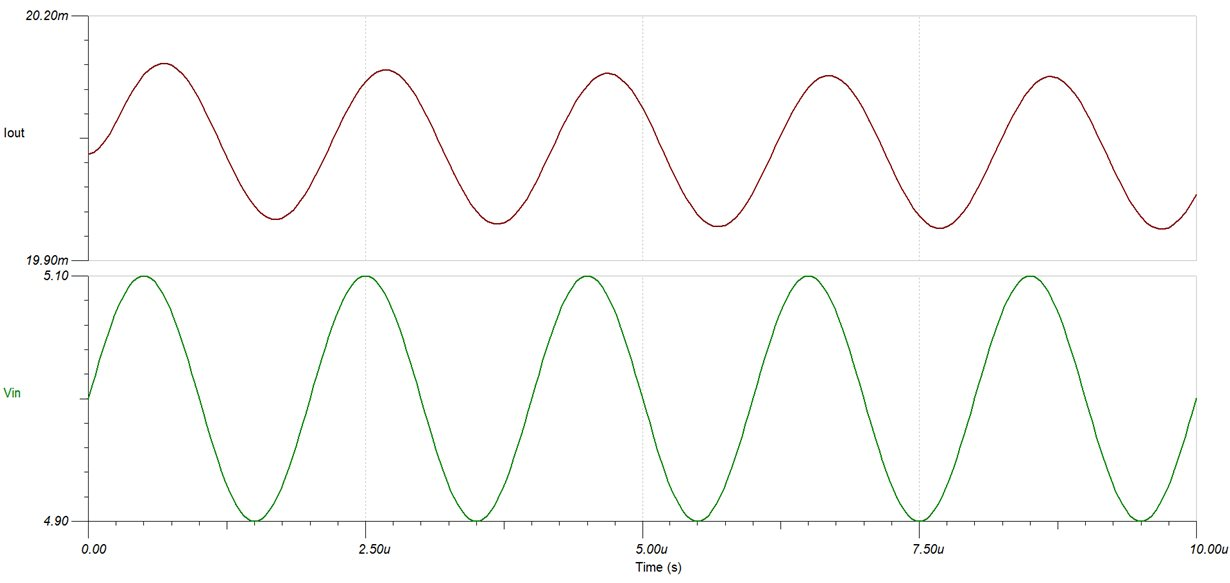When simulating the influence of PSRR on the above circuit,  adding a 100mV small sine wave in the power supply voltage source,  when the frequency of small sine wave is 50KHz, the wave of output current is 0.1mA and when the frequency is 500kHz,the wave of output current is under 0.6mA. The simulation results are shown as follow. Alough the output current wave increase with the increasing of frequency, and the trend is corresponding that PSRR decreases with increasing of frequncy, but the value is diffrent with theoretical calculation, why is that?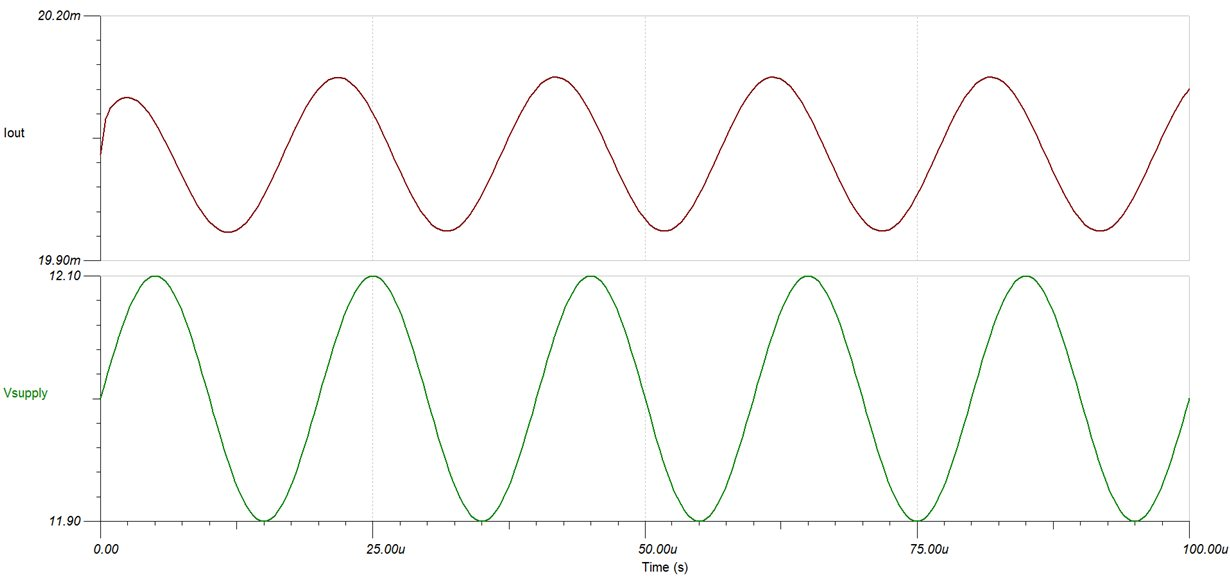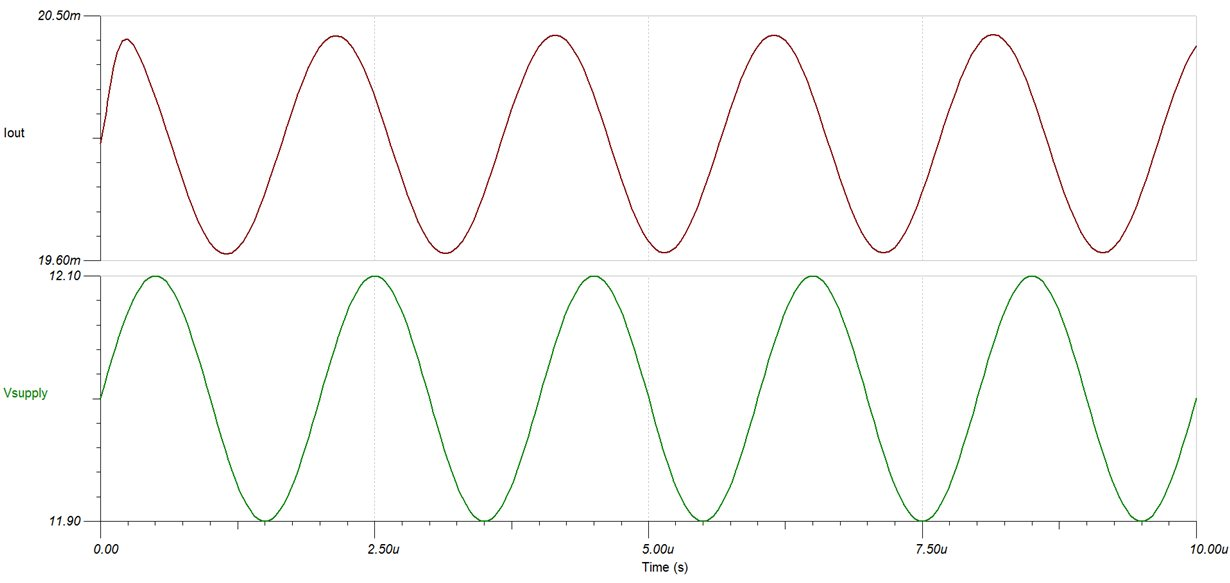• Hi,

None of your attachments/figures are visible.

Kindly reattach and resend this else we are not able to understand the issue.

• Hi Feng,

I recommend measuring the change in offset voltage due to a change in common mode voltage or supply voltage. By measuring the change in current due to a change in common mode or power supply (CMRR/PSRR) you need to include the V to I conversion which makes it slightly more difficult.

The offset voltage can be measured by placing a volt meter between the IN+ and IN- terminal of the op amp.

Also, as Ahana mentioned please repost the images or provide the TINA simulation file you are using.

Thank you,

Tim Claycomb

• Hi Feng,

Can you please try to post the images and attachments again? They did not come through in the original post.

Thank you,

Tim Claycomb

• Hi Tim,

The figures had been reattached.

Thank you for your help.

Best regards,

Feng Liu

• Hi Ahana,

The figures had been reattached.

Thank you for your help.

Best regards,

Feng Liu

• Hi Feng,

For your first question regarding CMRR the output is likely decreasing with an increase in frequency due to the bandwidth of the circuit. I recommend running an AC analysis on the circuit and see what the gain of the circuit is at 50kHz and 500kHz. If the gain is lower at 500kHz than 50kHz then this is the reason you are getting a lower amplitude.

For your second question regarding PSRR, remember that PSRR is the change in offset voltage due to a change in supply voltage. So if you measure the offset voltage, does the offset voltage match the specification of the device?

Thank you,

Tim Claycomb